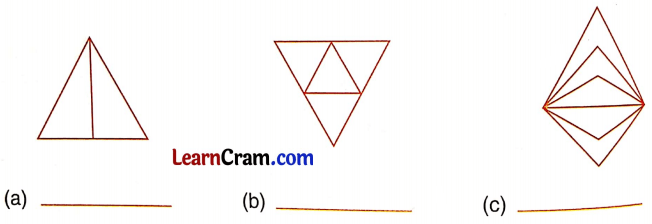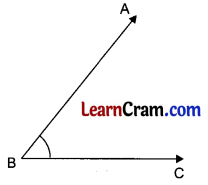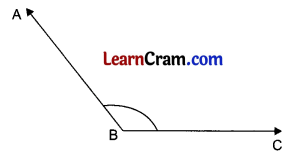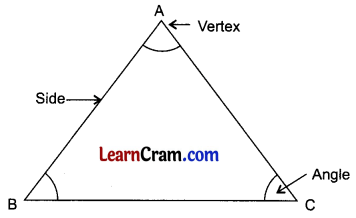# DAV Class 5 Maths Chapter 16 Worksheet 1 Solutions

The DAV Class 5 Maths Solutions and DAV Class 5 Maths Chapter 16 Worksheet 1 Solutions of Triangles offer comprehensive answers to textbook questions.

## DAV Class 5 Maths Ch 16 Worksheet 1 Solutions

Question 1.
How many vertices, sides, and angles does a triangle have?
Solution:
3 vertices, 3 sides, and 3 angles.

Question 2.
Draw a triangle. Name its vertices, sides, and angles.
Solution:A triangle has 3 vertices = A, B and C
A triangle has 3 sides = AB, BC, CA
A triangle has 3 angles = ∠BAC or ∠A, ∠CBA or ∠B, ∠ACB or ∠CQuestion 3.
You are given some triangles. Name its vertices, sides, and angles.Solution:Question 4.
Count and write how many triangles are there in each figure.Solution:
(a) 3
(b) 5
(c) 5

DAV Class 5 Maths Chapter 16 Worksheet 1 Notes

Triangle:
A triangle has three sides, three vertices, and three angles.

Line:
A line is an endless straight path that extends in both directions.
An infinite number of lines can be drawn to pass through a given point.

Acute Angle:
An angle that is less than 90° is called acute angle.Obtuse Angle:
An angle more than 90° but less than 180° is called obtuse angle.90° or Right Angle:
An angle that measures 90° is called a right angle.

Reflex Angle:
An angle that measures more than 180° is called a reflex angle.A triangle has three angles ∠BAC or ∠A, ∠CBA or ∠B, ∠ACB or ∠C
A triangle has 3 comers or 3 vertices – A, B and C.
A triangle has 3 sides – AB, BC, CA.